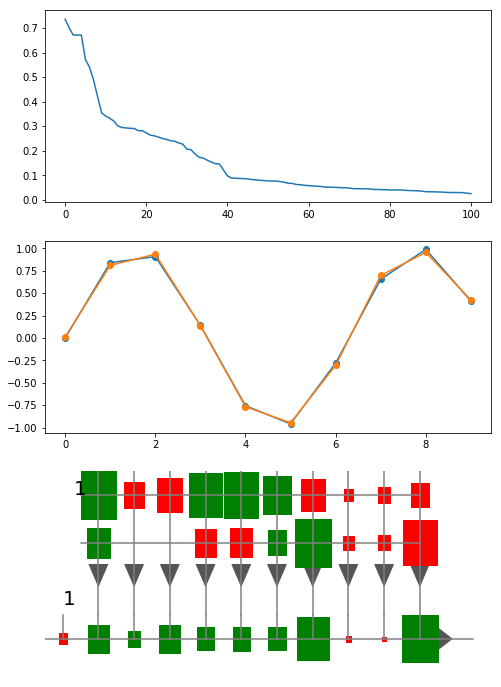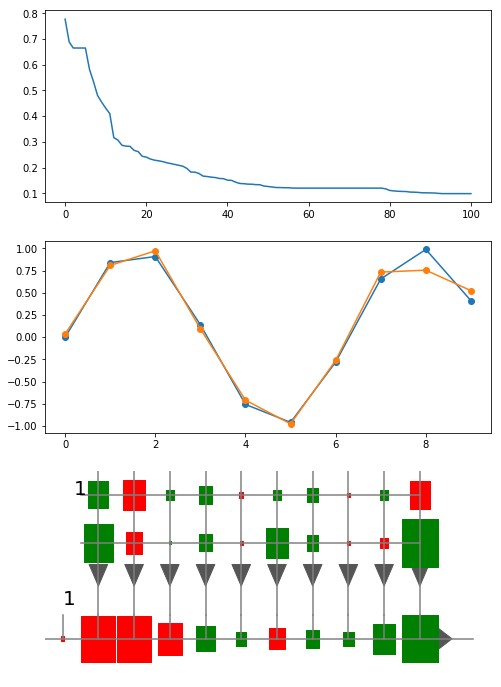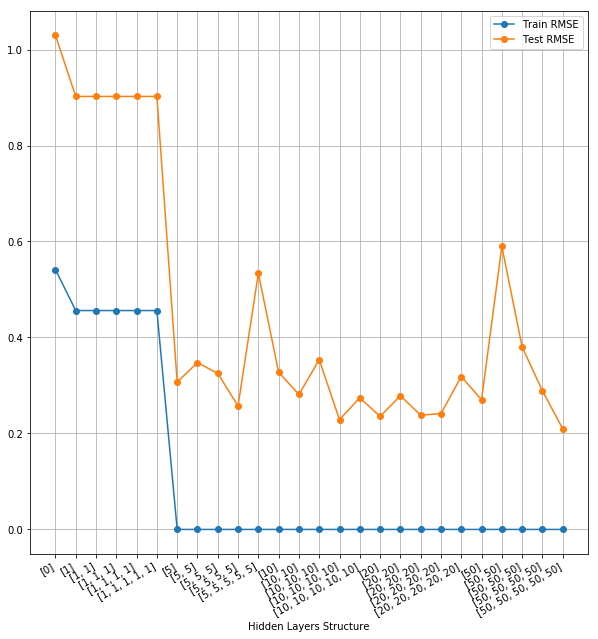$\newcommand{\xv}{\mathbf{x}} \newcommand{\Xv}{\mathbf{X}} \newcommand{\yv}{\mathbf{y}} \newcommand{\zv}{\mathbf{z}} \newcommand{\av}{\mathbf{a}} \newcommand{\Wv}{\mathbf{W}} \newcommand{\wv}{\mathbf{w}} \newcommand{\tv}{\mathbf{t}} \newcommand{\Tv}{\mathbf{T}} \newcommand{\muv}{\boldsymbol{\mu}} \newcommand{\sigmav}{\boldsymbol{\sigma}} \newcommand{\phiv}{\boldsymbol{\phi}} \newcommand{\Phiv}{\boldsymbol{\Phi}} \newcommand{\Sigmav}{\boldsymbol{\Sigma}} \newcommand{\Lambdav}{\boldsymbol{\Lambda}} \newcommand{\half}{\frac{1}{2}} \newcommand{\argmax}{\underset{#1}{\operatorname{argmax}}} \newcommand{\argmin}{\underset{#1}{\operatorname{argmin}}}$

# Assignment 3: Neural Network Regression with tanh and relu¶

Type your name here and rewrite all of the following sections. Add more sections to present your code, results, and discussions.

## Overview¶

The goal of this assignment is to

• gain some experience in comparing different sized neural networks when applied to a data set,
• implement a different activation function, relu, and compare with Tanh, and
• learn about object-oriented programming in python and to gain some experience in comparing different sized neural networks when applied to a data set.

Starting with the NeuralNetwork class from the lecture notes, you will create a new version of that class, apply it to a data set, and discuss the results. You will then create a second version, named NeuralNetwork_relu, that uses the relu activation function instead of the tanh function.

## Neural Network Code¶

Start with the NeuralNetwork class defined in lecture notes 09. Put that class definition as written into neuralnetworks.py into your current directory. Also place mlutilities.py from lecture notes 09 in your current directory. If this is done correctly, then the following code should run and produce results similar to what is shown here.

In :
import numpy as np
import matplotlib.pyplot as plt
%matplotlib inline

In :
import neuralnetworks as nn

X = np.arange(10).reshape((-1,1))
T = np.sin(X)

nnet = nn.NeuralNetwork(1, , 1)
nnet.train(X, T, 100, verbose=True)
nnet

SCG: Iteration 10 fValue 0.11650580464069538 Scale 0.003141839273812955
SCG: Iteration 20 fValue 0.07442533122854642 Scale 6.136404831665928e-06
SCG: Iteration 30 fValue 0.04266081411998552 Scale 5.9925828434237575e-09
SCG: Iteration 40 fValue 0.00963769976688828 Scale 1.1704263366062026e-11
SCG: Iteration 50 fValue 0.005973181139600653 Scale 1.1429944693419948e-14
SCG: Iteration 60 fValue 0.003402707338470135 Scale 1e-15
SCG: Iteration 70 fValue 0.0023506346299602054 Scale 1e-15
SCG: Iteration 80 fValue 0.0016538135439938544 Scale 1e-15
SCG: Iteration 90 fValue 0.001105639646154406 Scale 1e-15
SCG: Iteration 100 fValue 0.0006611417377810202 Scale 1e-15

Out:
NeuralNetwork(1, , 1)
Network was trained for 101 iterations that took 0.0420 seconds. Final error is 0.02571267659698267.
In :
plt.figure(figsize=(8, 12))
plt.subplot(3, 1, 1)
plt.plot(nnet.getErrors())

plt.subplot(3, 1, 2)
plt.plot(X, T, 'o-', label='Actual')
plt.plot(X, nnet.use(X), 'o-', label='Predicted')

plt.subplot(3, 1, 3)
nnet.draw()Now extract the parts of the neural network code that refer to the activation function and its derivative into two new methods. Modify the code in neuralnetworks.py by adding these two methods to the NeuralNetwork class:

def activation(self, weighted_sum):
return np.tanh(weighted_sum)

def activation_derivative(self, activation_value):
return 1 - activation_value * activation_value



Now replace the code in the appropriate places in the NeuralNetwork class so that np.tanh is replaced with a call to the self.activation method and its derivative is replaced by calls to self.activation_derivative. Tell jupyter to reload your changed code using the following lines.

In :
import imp
imp.reload(nn)

nnet = nn.NeuralNetwork(1, , 1)

In :
[nnet.activation(s) for s in [-2, -0.5, 0, 0.5, 2]]

Out:
[-0.9640275800758169,
-0.46211715726000974,
0.0,
0.46211715726000974,
0.9640275800758169]
In :
[nnet.activation_derivative(nnet.activation(s)) for s in [-2, -0.5, 0, 0.5, 2]]

Out:
[0.07065082485316443,
0.7864477329659274,
1.0,
0.7864477329659274,
0.07065082485316443]
In :
nnet.train(X, T, 100, verbose=True)
nnet

SCG: Iteration 10 fValue 0.18602791140657582 Scale 0.002693363969868201
SCG: Iteration 20 fValue 0.05804948574678485 Scale 2.6302382518244152e-06
SCG: Iteration 30 fValue 0.0388237474039478 Scale 2.5685920427972805e-09
SCG: Iteration 40 fValue 0.022956471354536757 Scale 5.0167813335884385e-12
SCG: Iteration 50 fValue 0.016096423417580375 Scale 4.8992005210824595e-15
SCG: Iteration 60 fValue 0.014504719989421952 Scale 1.6e-14
SCG: Iteration 70 fValue 0.014504719989421952 Scale 1.6777216e-08
SCG: Iteration 80 fValue 0.012417086856609912 Scale 0.004398046511104
SCG: Iteration 90 fValue 0.010361349819427426 Scale 4.294967296e-06
SCG: Iteration 100 fValue 0.009816566371544778 Scale 0.001099511627776

Out:
NeuralNetwork(1, , 1)
Network was trained for 101 iterations that took 0.0339 seconds. Final error is 0.09907858684672878.
In :
plt.figure(figsize=(8, 12))
plt.subplot(3, 1, 1)
plt.plot(nnet.getErrors())

plt.subplot(3, 1, 2)
plt.plot(X, T, 'o-', label='Actual')
plt.plot(X, nnet.use(X), 'o-', label='Predicted')

plt.subplot(3, 1, 3)
nnet.draw()## Neural Network Performance with Different Hidden Layer Structures and Numbers of Training Iterations¶

### Example with Toy Data¶

Using your new NeuralNetwork class, you can compare the error obtained on a given data set by looping over various hidden layer structures. Here is an example using the simple toy data from above.

In :
nRows = X.shape
rows = np.arange(nRows)
np.random.shuffle(rows)
nTrain = int(nRows * 0.8)
trainRows = rows[:nTrain]
testRows = rows[nTrain:]
Xtrain, Ttrain = X[trainRows, :], T[trainRows, :]
Xtest, Ttest = X[testRows, :], T[testRows, :]

In :
Xtrain.shape, Ttrain.shape, Xtest.shape, Ttest.shape

Out:
((8, 1), (8, 1), (2, 1), (2, 1))
In :
def rmse(A, B):
return np.sqrt(np.mean((A - B)**2))

In :
import pandas as pd

In :
def run_parameters(Xtrain, Ttrain, Xtest, Ttest, epochs_list, nh_list, verbose=True):

n_inputs = Xtrain.shape  # number of columns in X
n_outputs = Ttrain.shape  # number of columns in T

results = pd.DataFrame(columns=['Epochs', 'Hidden Units', 'RMSE Train', 'RMSE Test'])
for n_epochs in epochs_list:
for nh in nh_list:
nnet = nn.NeuralNetwork(Xtrain.shape, nh, n_outputs)
nnet.train(Xtrain, Ttrain, n_epochs)
Ytrain = nnet.use(Xtrain)
Ytest = nnet.use(Xtest)
results.loc[len(results)] = [n_epochs, nh, rmse(Ytrain, Ttrain),
rmse(Ytest, Ttest)]
if verbose:
display(results.tail(1))  # not print
return results

In :
results = run_parameters(Xtrain, Ttrain, Xtest, Ttest, [10, 100], [, , [10, 10]])

Epochs Hidden Units RMSE Train RMSE Test
0 10  0.541608 1.029781
Epochs Hidden Units RMSE Train RMSE Test
1 10  0.387781 0.38764
Epochs Hidden Units RMSE Train RMSE Test
2 10 [10, 10] 0.467083 0.815173
Epochs Hidden Units RMSE Train RMSE Test
3 100  0.541608 1.029781
Epochs Hidden Units RMSE Train RMSE Test
4 100  0.000005 0.341202
Epochs Hidden Units RMSE Train RMSE Test
5 100 [10, 10] 0.000425 0.372041
In :
results

Out:
Epochs Hidden Units RMSE Train RMSE Test
0 10  0.541608 1.029781
1 10  0.387781 0.387640
2 10 [10, 10] 0.467083 0.815173
3 100  0.541608 1.029781
4 100  0.000005 0.341202
5 100 [10, 10] 0.000425 0.372041
In :
hiddens = [] + [[nu] * nl for nu in [1, 5, 10, 20, 50] for nl in [1, 2, 3, 4, 5]]
hiddens

Out:
[,
,
[1, 1],
[1, 1, 1],
[1, 1, 1, 1],
[1, 1, 1, 1, 1],
,
[5, 5],
[5, 5, 5],
[5, 5, 5, 5],
[5, 5, 5, 5, 5],
,
[10, 10],
[10, 10, 10],
[10, 10, 10, 10],
[10, 10, 10, 10, 10],
,
[20, 20],
[20, 20, 20],
[20, 20, 20, 20],
[20, 20, 20, 20, 20],
,
[50, 50],
[50, 50, 50],
[50, 50, 50, 50],
[50, 50, 50, 50, 50]]
In :
results = run_parameters(Xtrain, Ttrain, Xtest, Ttest, , hiddens, verbose=False)
results

Out:
Epochs Hidden Units RMSE Train RMSE Test
0 500  5.416079e-01 1.029781
1 500  4.560940e-01 0.902303
2 500 [1, 1] 4.560869e-01 0.902301
3 500 [1, 1, 1] 4.560869e-01 0.902301
4 500 [1, 1, 1, 1] 4.560869e-01 0.902301
5 500 [1, 1, 1, 1, 1] 4.560869e-01 0.902301
6 500  2.008548e-09 0.307171
7 500 [5, 5] 2.090762e-09 0.347518
8 500 [5, 5, 5] 8.749549e-09 0.325134
9 500 [5, 5, 5, 5] 1.479444e-09 0.257220
10 500 [5, 5, 5, 5, 5] 3.943499e-09 0.533891
11 500  2.295153e-08 0.327571
12 500 [10, 10] 2.360269e-09 0.281235
13 500 [10, 10, 10] 4.246332e-09 0.353643
14 500 [10, 10, 10, 10] 2.900416e-09 0.228666
15 500 [10, 10, 10, 10, 10] 1.891290e-09 0.274473
16 500  2.606794e-08 0.235377
17 500 [20, 20] 2.472221e-09 0.278693
18 500 [20, 20, 20] 1.672955e-09 0.237996
19 500 [20, 20, 20, 20] 5.758939e-09 0.241380
20 500 [20, 20, 20, 20, 20] 3.070913e-09 0.318559
21 500  4.017171e-08 0.270330
22 500 [50, 50] 3.453968e-09 0.589909
23 500 [50, 50, 50] 5.530109e-09 0.380289
24 500 [50, 50, 50, 50] 5.181572e-09 0.288924
25 500 [50, 50, 50, 50, 50] 1.771950e-09 0.209846
In :
errors = np.array(results[['RMSE Train', 'RMSE Test']])
plt.figure(figsize=(10, 10))
plt.plot(errors, 'o-')
plt.legend(('Train RMSE', 'Test RMSE'))
plt.xticks(range(errors.shape), hiddens, rotation=30, horizontalalignment='right')
plt.xlabel('Hidden Layers Structure')
plt.grid(True)For this data (and the random shuffling of the data used when this notebook was run and random assignment of initial weight values), [20, 20, 20, 20, 20] produced the lowest test error.

Now, using the best hidden layer structure found, write the code that varies the number of training epochs and plot 'RMSE Train' and 'RMSE Test' versus the number of epochs.

### Experiments with Automobile Data¶

Now, repeat the above steps with the automobile mpg data we have used before. Set it up to use

• cylinders,
• displacement,
• weight,
• acceleration,
• year, and
• origin

as input variables, and

• mpg
• horsepower

as output variables.

## Experiments with relu activation function¶

Now define the NeuralNetwork_relu class by extending NeuralNetwork and simply redefine the activation and activation_derivative methods. Feel free to search the net for examples of how to define these functions. Try keywords like relu derivative python. Acknowledge the sites that you find helpful.

Your NeuralNetwork_relu class must be defined in your neuralnetworks.py file.

Write and run the code that repeats the above experiments with the auto-mpg data using NeuralNetwork_relu to evaluate different hidden layer structures and different numbers of epochs. To accomplish this, define a new function named run_parameters_act that has a new fifth argument to run_parameters called activation_functions that is passed a list with value ['tanh'], ['relu'], or ['tanh', 'relu'] to try both activation functions. In the body of run_parameters_act you must create and train the appropriate neural network based on the value of that parameter. The pandas DataFrame returned by run_parameters_act must include a column named 'Activation'.

Sort the results by the 'RMSE Test'. Pick one value of number of epochs that tends to produce the lowest 'RMSE Test' and select all rows of results for that number of epochs. Make one plot that shows the 'RMSE Test' versus hidden layer structure with one curve for 'tanh' and one curve for 'relu'. Use the plt.legend function to add a legend to the plot.

## Text descriptions¶

As always, discuss your results on the auto-mpg data. Discuss which hidden layer structures, numbers of iterations, and activation functions seem to do best. Your results will vary with different random partitions of the data. Investigate and discuss how much the best hidden layer structure and number of training iterations vary when you repeat the runs.

## Grading and Check-in¶

Your notebook will be run and graded automatically. Test this grading process by first downloading A3grader.tar and extract A3grader.py from it. Run the code in the following cell to demonstrate an example grading session. You should see a perfect execution score of 80 / 80 if your functions and class are defined correctly. The remaining 20 points will be based on the results you obtain from the comparisons of hidden layer structures and numbers of training iterations on the automobile data.

For the grading script to run correctly, you must first name this notebook as Lastname-A3.ipynb with Lastname being your last name, and then save this notebook. Define both neural network classes, NeuralNetwork and NeuralNetwork_relu in the file named neuralnetworks.py.

Combine your notebook and neuralnetwork.py into one zip file or tar file. Name your tar file Lastname-A3.tar or your zip file Lastname-A3.zip. Check in your tar or zip file using the Assignment 3 link in Canvas.

A different, but similar, grading script will be used to grade your checked-in notebook. It will include other tests.

In :
%run -i A3grader.py

======================= Code Execution =======================

Extracting python code from notebook named 'A3 Neural Network Regression and Activation Functions.ipynb' and storing in notebookcode.py
Removing all statements that are not function or class defs or import statements.

Testing:
import neuralnetworks as nn
nnet = nn.NeuralNetwork(4, , 1)
acts = nnet.activation(np.array([-0.5, 1.5]))

--- 10/10 points. nnet.activation() is correct.

Testing:
dacts = nnet.activation_derivative([-0.46211716  0.90514825])

--- 10/10 points. nnet.activation_derivative() is correct.

Testing:
import neuralnetworks as nn
nnet_relu = nn.NeuralNetwork_relu(4, , 1)
acts = nnet_reul.activation(np.array([-0.5, 1.5]))

--- 10/10 points. nnet.activation() is correct.

Testing:
dacts = nnet_relu.activation_derivative([0.  1.5])

--- 10/10 points. nnet.activation_derivative() is correct.

Testing:
data = np.loadtxt('machine.data', delimiter=',', usecols=range(2, 10))
X = data[:, :4]
T = data[:, -2:]
Xtrain = X[20:60, :]
Ttrain = T[20:60, :]
Xtest = X[70:100:, :]
Ttest = T[70:100:, :]

results = run_parameters_act(Xtrain, Ttrain, Xtest, Ttest, ['tanh', 'relu'],
[1, 1000], [, ], verbose=False)
results = results.sort_values('RMSE Test')
print(results)
Activation Epochs Hidden Units  RMSE Train   RMSE Test
5       relu      1           28.957669   36.764358
6       relu   1000             26.464877   41.154890
2       tanh   1000             18.294730   62.210623
1       tanh      1           38.964274   86.443440
4       relu      1             52.962688  110.584275
7       relu   1000           11.219595  132.671880
0       tanh      1             61.989319  136.147861
3       tanh   1000            8.033079  176.129114

--- 40/40 points. Best activation function is correctly 'relu'

A3 Execution Grade is 80 / 80

Remaining 20 points will be based on your text descriptions of results and plots.

A3 FINAL GRADE is   / 100

A3 EXTRA CREDIT is   / 1


## Extra Credit¶

Create yet another version of the neural network class, called NeuralNetwork_logistic, that uses the logistic activation function and its derivative, and repeat the above comparisons.